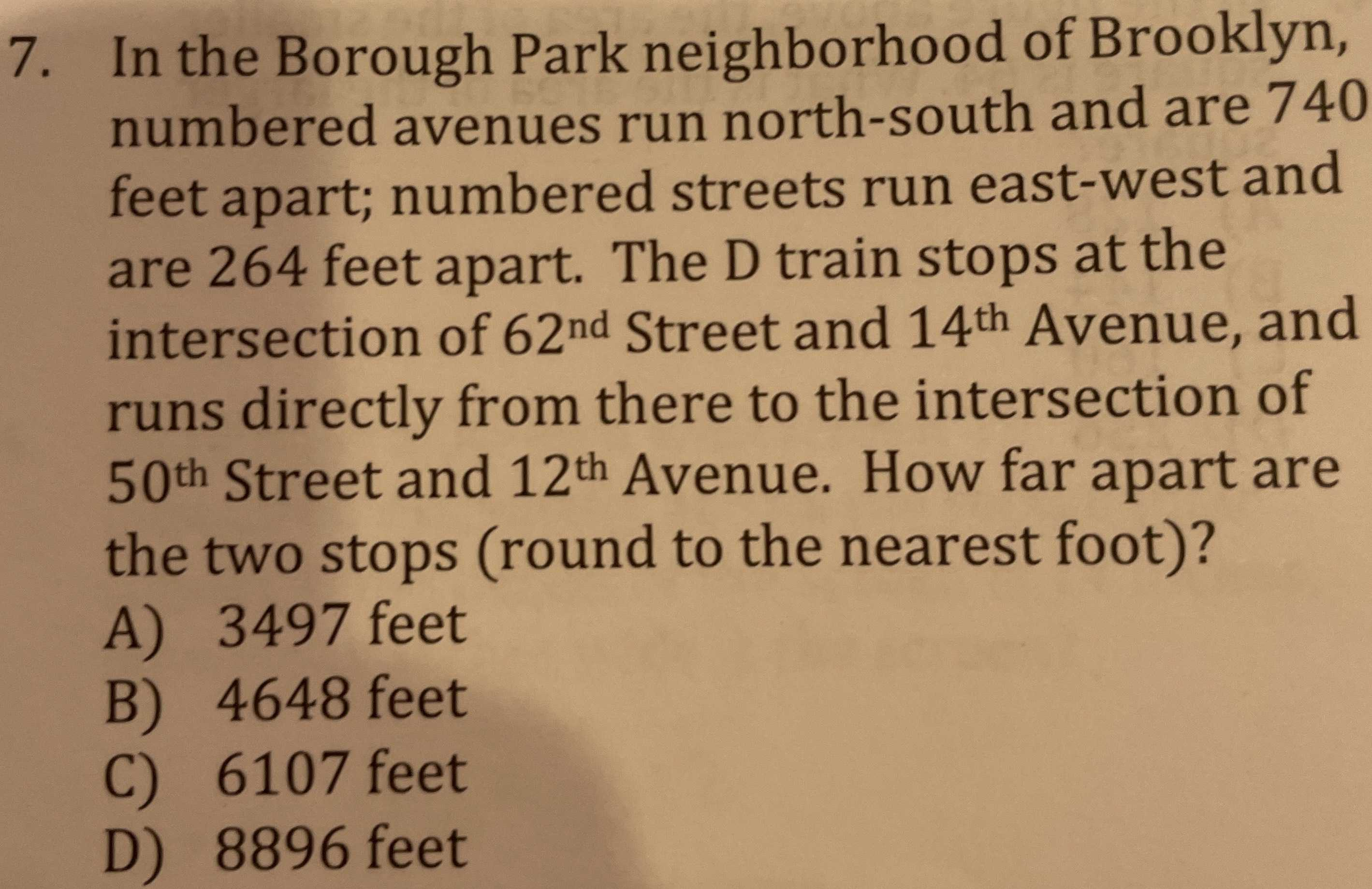### Still have math questions?

Arithmetic
Question7. In the Borough Park neighborhood of Brooklyn, numbered avenues run north-south and are $$740$$ feet apart; numbered streets run east-west and are $$264$$ feet apart. The D train stops at the intersection of 62nd Street and 14th Avenue, and runs directly from there to the intersection of

50th Street and 12th Avenue. How far apart are the two stops (round to the nearest foot)? A) $$3497$$ feet B) $$4648$$ feet C) $$6107$$ feet D) $$8896$$ feet